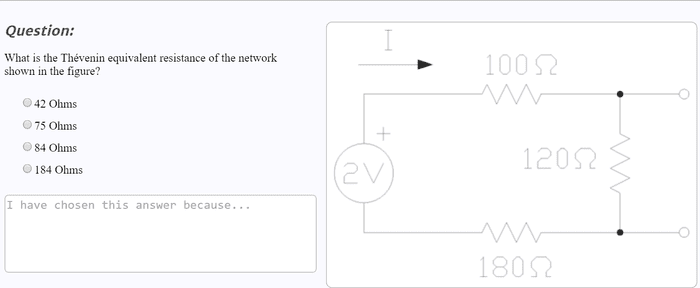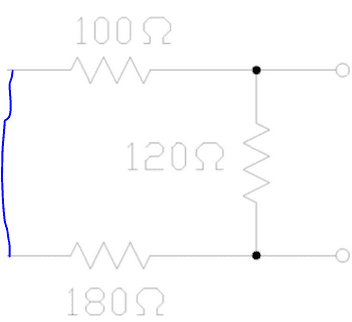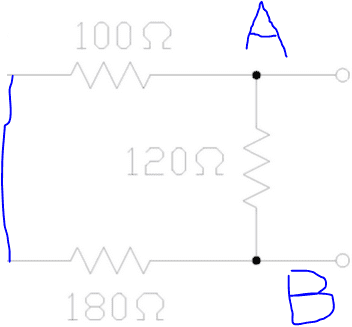# Thevenin equivalent resistance problem

## Homework StatementV=IR

## The Attempt at a Solution

[/B]
I found that the current around the circuit was 5mA using kirchoffs law ( i think)
If this is true then the T.E.V should be the voltage across the 120ohm resistor which is .6V (120*5e-3). Now i'm stuck and not sure how to find the T.E.R, thankyou

SammyS
Staff Emeritus
Homework Helper
Gold Member

## Homework Statement

[ IMG]http://i.imgur.com/XUot0kO.png[/PLAIN]

V=IR

## The Attempt at a Solution

I found that the current around the circuit was 5mA using kirchoffs law ( i think)
If this is true then the T.E.V should be the voltage across the 120ohm resistor which is .6V (120*5e-3). Now i'm stuck and not sure how to find the T.E.R, thankyou[/B]
Hello glover261. Welcome to PF !

So far, so good.

What have you been taught regarding how to find the Thevenin equivalent resistance ?

Hello glover261. Welcome to PF !

So far, so good.

What have you been taught regarding how to find the Thevenin equivalent resistance ?
Hi thanks, I thought you were supposed to take out all components except resistors in the circuit, current sources by open circuit and voltage sources through short circuit. Then just add up the total resistance of all the resistors, but that method does not seem to work on this problem, as none of the answers are the total resistance.

SammyS
Staff Emeritus
Homework Helper
Gold Member
Hi thanks, I thought you were supposed to take out all components except resistors in the circuit, current sources by open circuit and voltage sources through short circuit. Then just add up the total resistance of all the resistors, but that method does not seem to work on this problem, as none of the answers are the total resistance.
That's not quite right. You don't simply add all the resistances. You find the equivalent resistance across the terminals.

phinds
Gold Member
2021 Award
Hi thanks, I thought you were supposed to take out all components except resistors in the circuit, current sources by open circuit and voltage sources through short circuit. Then just add up the total resistance of all the resistors, but that method does not seem to work on this problem, as none of the answers are the total resistance.
Is "adding up the total resistance of all the resistors" really what happens in a circuit? Do parallel resistors add?

EDIT: I see sammy beat me to it.

Aren't they all in series though? or am i missing something

SammyS
Staff Emeritus
Homework Helper
Gold Member
Aren't they all in series though? or am i missing something
You must be missing something.

Draw what you have when the voltage source is shorted.phinds
Gold Member
2021 Award
Aren't they all in series though? or am i missing something
Probably be a good idea to go back to basics and study what parallel and series really look like. This is a very common difficulty in beginning study of circuits so it's best to get really solid with it in the beginning.

EDIT: dammit, sammy, you're too fast for meYou must be missing something.

Draw what you have when the voltage source is shorted.

View attachment 99280

Sorry I still can't see it

phinds
Gold Member
2021 Award
Sorry I still can't see it
Probably be a good idea to go back to basics and study what parallel and series really look like. This is a very common difficulty in beginning study of circuits so it's best to get really solid with it in the beginning.

SammyS
Staff Emeritus
Homework Helper
Gold Member
Sorry I still can't see it
See the terminals?

(phinds beat me this time !)

See the terminals?

(phinds beat me this time !)

So, the 180 and 100 resistors are in parallel. So Rt = 1/((1/180)+(1/100)) + 120 = 184 ohms? Is that right because I thought for a pair of components to be in parallel, both sides had to be connected to the same node.

SammyS
Staff Emeritus
Homework Helper
Gold Member
So, the 180 and 100 resistors are in parallel. No.

So Rt = 1/((1/180)+(1/100)) + 120 = 184 ohms?

Is that right because

I thought for a pair of components to be in parallel, both sides had to be connected to the same node. This is correct.
See the terminals?

See the terminals?

What do they mean?

SammyS
Staff Emeritus
Homework Helper
Gold Member
What do they mean?
Find the equivalent resistance across the terminals.

Look at the nodes again.SammyS
Staff Emeritus
Homework Helper
Gold Member
There is an alternate way to find the Thevenin resistance.

Take the original circuit, and find the current which flows through a wire which is connected to the two terminals. (This is the short circuit current.)

This is the same current which will flow through the Thevenin equivalent circuit if its terminals are shorted.

In other words, this current times the Thenenin resistance is equal to the Thevenin voltage.